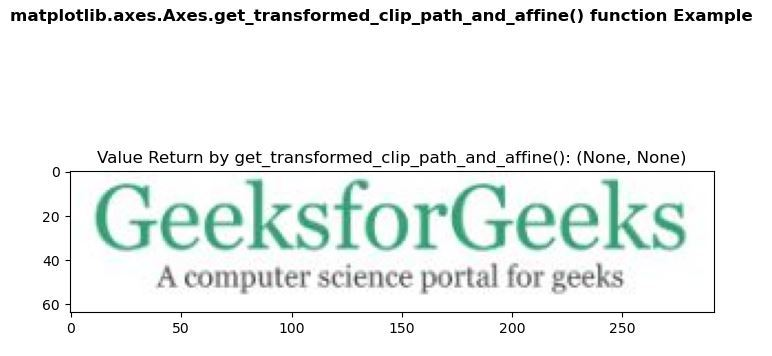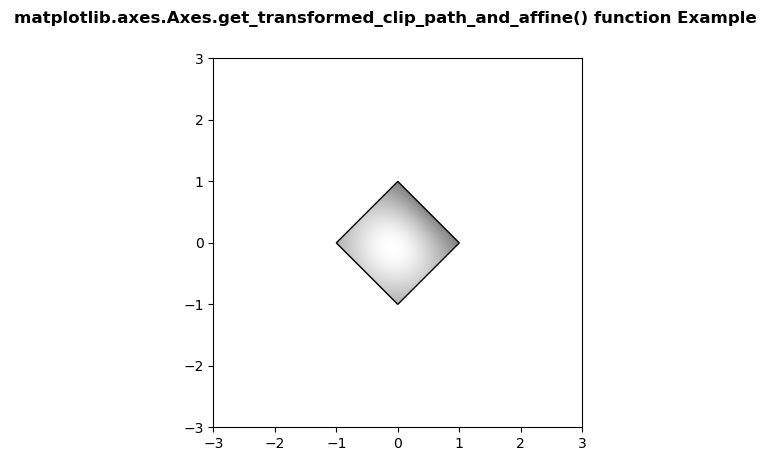Related Articles
Matplotlib.axes.Axes.get_transformed_clip_path_and_affine() in Python
• Last Updated : 30 Apr, 2020

Matplotlib is a library in Python and it is numerical – mathematical extension for NumPy library. The Axes Class contains most of the figure elements: Axis, Tick, Line2D, Text, Polygon, etc., and sets the coordinate system. And the instances of Axes supports callbacks through a callbacks attribute.

## matplotlib.axes.Axes.get_transformed_clip_path_and_affine() Function

The Axes.get_transformed_clip_path_and_affine() function in axes module of matplotlib library is used to get the clip path with the non-affine part of its transformation applied, and the remaining affine part of its transformation.

Syntax: Axes.get_transformed_clip_path_and_affine(self)

Parameters: This method does not accepts any parameter.

Returns: This method return the clip path with the non-affine part of its transformation applied, and the remaining affine part of its transformation.

Below examples illustrate the matplotlib.axes.Axes.get_transformed_clip_path_and_affine() function in matplotlib.axes:

Example 1:

Image used:`# Implementation of matplotlib function``import` `matplotlib.pyplot as plt``import` `matplotlib.patches as patches``import` `matplotlib.cbook as cbook``    ` ` ` `with cbook.get_sample_data(``'loggf.PNG'``) as image_file:``    ``image ``=` `plt.imread(image_file)``    ` `fig, ax ``=` `plt.subplots()``im ``=` `ax.imshow(image)``patch ``=` `patches.Rectangle((``0``, ``0``), ``260``, ``200``, ``                          ``transform ``=` `ax.transData)``  ` `ax.set_title(``"Value Return by get_transformed_clip_path_and_affine(): "``             ``+``str``(im.get_transformed_clip_path_and_affine()))``      ` `fig.suptitle('matplotlib.axes.Axes.get_transformed_clip_path_and_affine()\`` ``function Example\n\n', fontweight ``=``"bold"``)`` ` `plt.show()`

Output:Example 2:

 `# Implementation of matplotlib function``import` `numpy as np``import` `matplotlib.cm as cm``import` `matplotlib.pyplot as plt``from` `matplotlib.path ``import` `Path``from` `matplotlib.patches ``import` `PathPatch``    ` ` ` `delta ``=` `0.025`` ` `x ``=` `y ``=` `np.arange(``-``3.0``, ``3.0``, delta)``X, Y ``=` `np.meshgrid(x, y)`` ` `Z1 ``=` `np.exp(``-``X``*``*``2` `-` `Y``*``*``2``)``Z2 ``=` `np.exp(``-``(X ``-` `1``)``*``*``2` `-` `(Y ``-` `1``)``*``*``2``)``Z ``=` `(Z1 ``-` `Z2) ``*` `2``    ` `path ``=` `Path([[``0``, ``1``], [``1``, ``0``], [``0``, ``-``1``], [``-``1``, ``0``], [``0``, ``1``]])``patch ``=` `PathPatch(path, facecolor ``=``'none'``)``    ` `fig, ax ``=` `plt.subplots()``ax.add_patch(patch)``im ``=` `ax.imshow(Z,``               ``interpolation ``=``'bilinear'``, ``               ``cmap ``=` `cm.gray,``               ``origin ``=``'lower'``,``               ``extent ``=``[``-``3``, ``3``, ``-``3``, ``3``],``               ``clip_path ``=` `patch,``               ``clip_on ``=` `True``)`` ` `print``(``"Value Return by get_transformed_clip_path_and_affine(): "``)`` ` `for` `i ``in` `im.get_transformed_clip_path_and_affine():``    ``print``(i)``      ` `fig.suptitle('matplotlib.axes.Axes.get_transformed_clip_path_and_affine()\`` ``function Example\n\n', fontweight ``=``"bold"``)`` ` `plt.show()`

Output:```Value Return by get_transformed_clip_path_and_affine():
Path(array([[ 0.,  1.],
[ 1.,  0.],
[ 0., -1.],
[-1.,  0.],
[ 0.,  1.]]), None)
Affine2D(
[[ 82.66666667   0.         328.        ]
[  0.          61.6        237.6       ]
[  0.           0.           1.        ]])
```

Attention geek! Strengthen your foundations with the Python Programming Foundation Course and learn the basics.

To begin with, your interview preparations Enhance your Data Structures concepts with the Python DS Course. And to begin with your Machine Learning Journey, join the Machine Learning – Basic Level Course

My Personal Notes arrow_drop_up